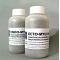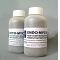##### The Japanese Bonsai specialist
Direct order Contact Help / Services Newsletter# Endo and ecto mycorhize bonsai treatment twin pack

› Bonsai soil and fertiliser › Bonsai maintenanceref. : 6235

20,00

Available quantity : 5Order

###### Description

Two-pack, one 100g bottle of ECTO mycorhize, and one 100g bottle of ENDO mycorhize.

ECTO mycorhize is for willow, hazelnut catkin trees, walnut, alder, birch, beech, fir, pine, cedar, larch, spruce, chestnut, myrtle, poplar, eucalyptus and oak.

ENDO mycorhize is for fertilising orchids, maple, juniper, ash, elm, fruit and tropical bonsai trees.

It's especially good for bonsai with root problems such as excess water or root rot. It's highly recommended that you use this product when potting trees found in the forest (yamadori) that you want to train into a bonsai.

Benefits: mycorhize may reduce infection by bacteria and fungi that cause plant diseases. It can also help fix vital nutrients, promoting plant growth.

How to use: Use 1 to 3 capfuls per tree, make four or five holes in the earth, near the trunk and surface roots (not in the bottom of the pot), and add some powder to each. Re-cover the holes with earth afterwards.

#mycorhize 7.9 #bonsai 7.6 #ecto 4.4 #endo 4.4 #trees 3.5 #pack 3.4 #that 3.4 #maintenance 3.1 #treatment 2.9 #bottle 2.6

Formule
(( ROUND((CHAR_LENGTH(b.article_nom)-CHAR_LENGTH(REPLACE(b.article_nom, 'mycorhize', '')))/LENGTH('mycorhize')) + ROUND((CHAR_LENGTH(b.article_description)-CHAR_LENGTH(REPLACE(b.article_description, 'mycorhize', '')))/LENGTH('mycorhize')) ) * 7.9) + (( ROUND((CHAR_LENGTH(b.article_nom)-CHAR_LENGTH(REPLACE(b.article_nom, 'bonsai', '')))/LENGTH('bonsai')) + ROUND((CHAR_LENGTH(b.article_description)-CHAR_LENGTH(REPLACE(b.article_description, 'bonsai', '')))/LENGTH('bonsai')) ) * 5.6) + (( ROUND((CHAR_LENGTH(b.article_nom)-CHAR_LENGTH(REPLACE(b.article_nom, 'endo', '')))/LENGTH('endo')) + ROUND((CHAR_LENGTH(b.article_description)-CHAR_LENGTH(REPLACE(b.article_description, 'endo', '')))/LENGTH('endo')) ) * 4.4) + (( ROUND((CHAR_LENGTH(b.article_nom)-CHAR_LENGTH(REPLACE(b.article_nom, 'ecto', '')))/LENGTH('ecto')) + ROUND((CHAR_LENGTH(b.article_description)-CHAR_LENGTH(REPLACE(b.article_description, 'ecto', '')))/LENGTH('ecto')) ) * 4.4) + (( ROUND((CHAR_LENGTH(b.article_nom)-CHAR_LENGTH(REPLACE(b.article_nom, 'trees', '')))/LENGTH('trees')) + ROUND((CHAR_LENGTH(b.article_description)-CHAR_LENGTH(REPLACE(b.article_description, 'trees', '')))/LENGTH('trees')) ) * 3.5) + (( ROUND((CHAR_LENGTH(b.article_nom)-CHAR_LENGTH(REPLACE(b.article_nom, 'that', '')))/LENGTH('that')) + ROUND((CHAR_LENGTH(b.article_description)-CHAR_LENGTH(REPLACE(b.article_description, 'that', '')))/LENGTH('that')) ) * 3.4) + (( ROUND((CHAR_LENGTH(b.article_nom)-CHAR_LENGTH(REPLACE(b.article_nom, 'pack', '')))/LENGTH('pack')) + ROUND((CHAR_LENGTH(b.article_description)-CHAR_LENGTH(REPLACE(b.article_description, 'pack', '')))/LENGTH('pack')) ) * 3.4) + (( ROUND((CHAR_LENGTH(b.article_nom)-CHAR_LENGTH(REPLACE(b.article_nom, 'treatment', '')))/LENGTH('treatment')) + ROUND((CHAR_LENGTH(b.article_description)-CHAR_LENGTH(REPLACE(b.article_description, 'treatment', '')))/LENGTH('treatment')) ) * 2.9) + (( ROUND((CHAR_LENGTH(b.article_nom)-CHAR_LENGTH(REPLACE(b.article_nom, 'bottle', '')))/LENGTH('bottle')) + ROUND((CHAR_LENGTH(b.article_description)-CHAR_LENGTH(REPLACE(b.article_description, 'bottle', '')))/LENGTH('bottle')) ) * 2.6) + (( ROUND((CHAR_LENGTH(b.article_nom)-CHAR_LENGTH(REPLACE(b.article_nom, 'plant', '')))/LENGTH('plant')) + ROUND((CHAR_LENGTH(b.article_description)-CHAR_LENGTH(REPLACE(b.article_description, 'plant', '')))/LENGTH('plant')) ) * 2.5)

## Secure payment## Delivery

Our logistic partners :04 74 55 23 48
Pépinière MAILLOT-BONSAÏ
Le Bois Frazy
01990 RELEVANT - FRANCE
on appointment